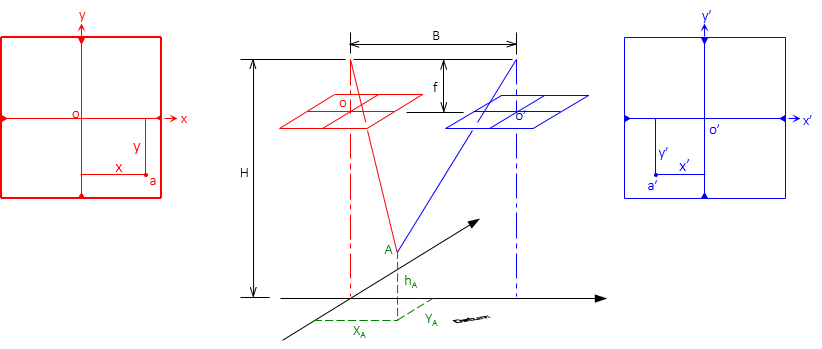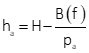## 3. Parallax Equations

### a. Ground Coordinates

The ground coordinate system is similar to that of a single vertical photo. The origin and axes directions are defined by the left photo, Figure E-9, using the actual flight direction.Figure E-9Ground Coordinate System

Coordinates and elevation of ground points can be determined from parallax measurements using equations E-4 through E-6.Equation E-4Equation E-5Equation E-6

### b. Elevations by Parallax

Figure E-10 shows the basic parallax and elevation geometry for two points.Figure E-10Parallax and Elevation Difference

If we can measure the parallax of both points, we can determine one point's elevation based on the other, Equation E-7.Equation E-7